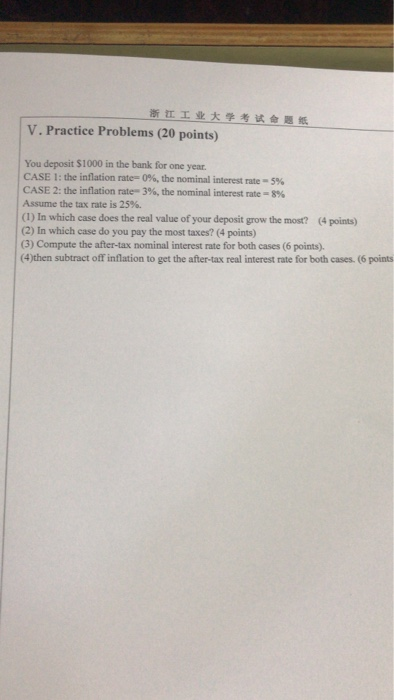### Create an Account

Home / Questions / 浙江工业大学考试会題纸 V. Practice Problems (20 points) You de

# 浙江工业大学考试会題纸 V. Practice Problems (20 points) You deShow transcribed image text浙江工业大学考试会題纸 V. Practice Problems (20 points) You deposit \$1000 in the bank for one year. CASE 1: the inflation rate=0%, the nominal interest rate -5% CASE 2: the inflation rate-3%, the nominal interest rate=8% Assume the tax rate is 25%. (1) In which case does the real value of your deposit grow the most? (4 points) (2) In which case do you pay the most taxes? (4 points) (3) Compute the after-tax nominal interest rate for both cases (6 points). (4)then subtract off inflation to get the after-tax real interest rate for both cases. (6 points

Aug 14 2020 View more View LessSubscribe To Get Solution